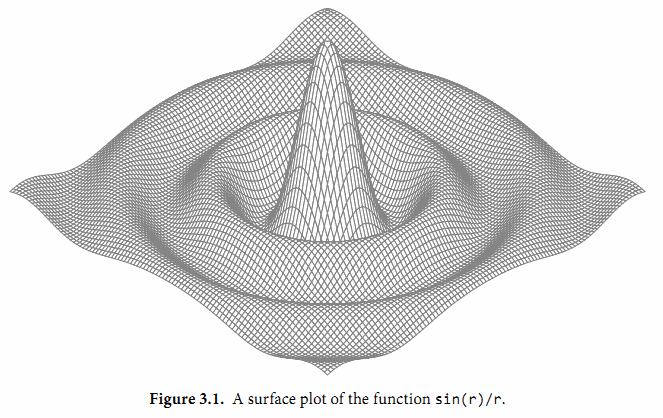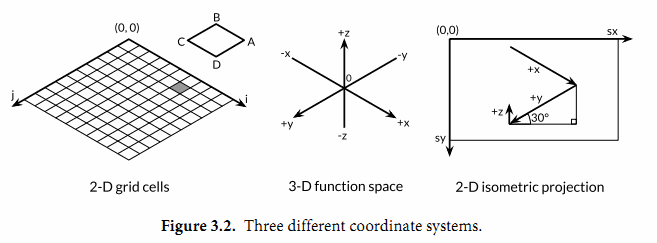## 3.2. 浮點數

Go語言提供了兩種精度的浮點數，float32和float64。它們的算術規范由IEEE754浮點數国際標準定義，該浮點數規范被所有現代的CPU支持。

var f float32 = 16777216 // 1 << 24
fmt.Println(f == f+1)    // "true"!


const e = 2.71828 // (approximately)


const Avogadro = 6.02214129e23  // 阿伏伽德羅常數
const Planck   = 6.62606957e-34 // 普朗剋常數


for x := 0; x < 8; x++ {
fmt.Printf("x = %d e^x = %8.3f\n", x, math.Exp(float64(x)))
}


x = 0       e^x =    1.000
x = 1       e^x =    2.718
x = 2       e^x =    7.389
x = 3       e^x =   20.086
x = 4       e^x =   54.598
x = 5       e^x =  148.413
x = 6       e^x =  403.429
x = 7       e^x = 1096.633


math包中除了提供大量常用的數學函數外，還提供了IEEE754浮點數標準中定義的特殊值的創建和測試：正無窮大和負無窮大，分别用於表示太大溢出的數字和除零的結果；還有NaN非數，一般用於表示無效的除法操作結果0/0或Sqrt(-1).

var z float64
fmt.Println(z, -z, 1/z, -1/z, z/z) // "0 -0 +Inf -Inf NaN"


nan := math.NaN()
fmt.Println(nan == nan, nan < nan, nan > nan) // "false false false"


func compute() (value float64, ok bool) {
// ...
if failed {
return 0, false
}
return result, true
}gopl.io/ch3/surface
// Surface computes an SVG rendering of a 3-D surface function.
package main

import (
"fmt"
"math"
)

const (
width, height = 600, 320            // canvas size in pixels
cells         = 100                 // number of grid cells
xyrange       = 30.0                // axis ranges (-xyrange..+xyrange)
xyscale       = width / 2 / xyrange // pixels per x or y unit
zscale        = height * 0.4        // pixels per z unit
angle         = math.Pi / 6         // angle of x, y axes (=30°)
)

var sin30, cos30 = math.Sin(angle), math.Cos(angle) // sin(30°), cos(30°)

func main() {
fmt.Printf("<svg xmlns='http://www.w3.org/2000/svg' "+
"style='stroke: grey; fill: white; stroke-width: 0.7' "+
"width='%d' height='%d'>", width, height)
for i := 0; i < cells; i++ {
for j := 0; j < cells; j++ {
ax, ay := corner(i+1, j)
bx, by := corner(i, j)
cx, cy := corner(i, j+1)
dx, dy := corner(i+1, j+1)
fmt.Printf("<polygon points='%g,%g %g,%g %g,%g %g,%g'/>\n",
ax, ay, bx, by, cx, cy, dx, dy)
}
}
fmt.Println("</svg>")
}

func corner(i, j int) (float64, float64) {
// Find point (x,y) at corner of cell (i,j).
x := xyrange * (float64(i)/cells - 0.5)
y := xyrange * (float64(j)/cells - 0.5)

// Compute surface height z.
z := f(x, y)

// Project (x,y,z) isometrically onto 2-D SVG canvas (sx,sy).
sx := width/2 + (x-y)*cos30*xyscale
sy := height/2 + (x+y)*sin30*xyscale - z*zscale
return sx, sy
}

func f(x, y float64) float64 {
r := math.Hypot(x, y) // distance from (0,0)
return math.Sin(r) / r
}(x,y,z)投影到二維的畵布中。畵布中從遠處到右邊的點對應較大的x值和較大的y值。併且畵布中x和y值越大，則對應的z值越小。x和y的垂直和水平縮放繫數來自30度角的正絃和餘絃值。z的縮放繫數0.4，是一個任意選擇的參數。

w.Header().Set("Content-Type", "image/svg+xml")


（這一步在Lissajous例子中不是必鬚的，因爲服務器使用標準的PNG圖像格式，可以根據前面的512個字節自動輸出對應的頭部。）允許客戶端通過HTTP請求參數設置高度、寬度和顔色等參數。